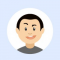## Write a Golang program to find the sum of digits for a given numberPublished on 04-Feb-2021 10:57:15
Examplesnum = 125 => 1 + 2 + 5 = 8num = 101 => 1 + 0 + 1 = 2num = 151 => 1 + 5 + 1 = 7Approach to solve this problemStep 1: Define a function that accepts numbers(num); type is int.Step 2: Start a true loop ... Read More

## Write a Golang program to check whether a given number is a palindrome or notPublished on 04-Feb-2021 10:56:39
Definition: A palindrome is a number which is similar when read from the front and from the rear.Examplesnum = 121 => Palindromenum = 13131 => Palindromenum = 123 => Not a PalindromeApproach to solve this problemStep 1: Define a function that accepts a numbers(num); type is int.Step 2: Start making the ... Read More

## Write a Golang program to print the Fibonacci seriesPublished on 04-Feb-2021 10:56:00
Definition: In a Fibonacci series, the next number would be the summation of its two previous numbers, series starting from 0 and 1.ExamplesPrint a fibonacci series up to num = 10;Series: 1, 2, 3, 5, 8, next is 13 but greater than 10;Approach to solve this problemStep 1: Define a function ... Read More

## Write a Golang program to swap two numbers without using a third variablePublished on 04-Feb-2021 10:53:45
Approach to solve this problemStep 1: Define a function that accepts two numbers, type is int.Step 2: Find b = a + b;Step 3: Then a = b – a and b = b – aProgramLive Demopackage main import "fmt" func swap(a, b int){    fmt.Printf("Before swapping, numbers are ... Read More

## Write a Golang program to find the factorial of a given number (Using Recursion)Published on 04-Feb-2021 10:53:10
ExamplesFactorial of 5 = 5*4*3*2*1 = 120Factorial of 10 = 10*9*8*7*6*5*4*3*2*1 =Approach to solve this problemStep 1: Define a function that accepts a number (greater than 0), type is int.Step 2: If the number is 1, then return 1.Step 3: Otherwise, return num*function(num-1).ProgramLive Demopackage main import "fmt" func factorial(num int) ... Read More

## Write a Golang program to search an element in an arrayPublished on 04-Feb-2021 10:51:40
Definition: A number is that is greater than 2 and divisible only by itself and 1.Examples: Prime numbers are 2, 3, 5, 7, 11, 13, 113, 119, ..., etc.Approach to solve this problemStep 1: Find square root of the given number, sq_root = √numStep 2: If the given number is divisible by ... Read More

## Write a Golang program to check whether a given number is prime number or notPublished on 04-Feb-2021 10:49:32
Definition: A number is that is greater than 2 and divisible only by itself and 1.Examples: Prime numbers are 2, 3, 5, 7, 11, 13, 113, 119, ..., etc.Approach to solve this problemStep 1: Find square root of the given number, sq_root = √numStep 2: If the given number is divisible by ... Read More

## Write a Golang program to find the element with the minimum value in an arrayPublished on 04-Feb-2021 10:48:03
ExamplesA1 = [2, 4, 6, 7, 8, 10, 3, 6, 0, 1]; Minimum number is 0;A2 = [12, 14, 16, 17, 18, 110, 13, 16, 10, 11]; Minimum number is 10;Approach to solve this problemStep 1: Consider the number at the 0th index as the minimum number, min_num = A.Step ... Read More

## Write a program in Go language to find the element with the maximum value in an arrayPublished on 04-Feb-2021 10:46:55
ExamplesA1 = [2, 4, 6, 7, 8, 10, 3, 6, 0, 1]; Maximum number is 10A2 = [12, 14, 16, 17, 18, 110, 13, 16, 10, 11]; Maximum number is 110Approach to solve this problemStep 1: Consider the number at the 0th index as the maximum number, max_num = AStep 2: Compare ... Read More

## How to display open cursors in Oracle?Published on 05-Dec-2020 06:25:08
Problem:You want to display open cursors in Oracle.SolutionWe can query the data dictionary to determine the number of cursors that are open per session. "V\$SESSION" provides a more accurate number of the cursors currently open than "V\$OPEN_CURSOR".Exampleselect  a.value  , c.username  , c.machine  , c.sid  , c.serial# from v\$sesstat a  , ... Read More